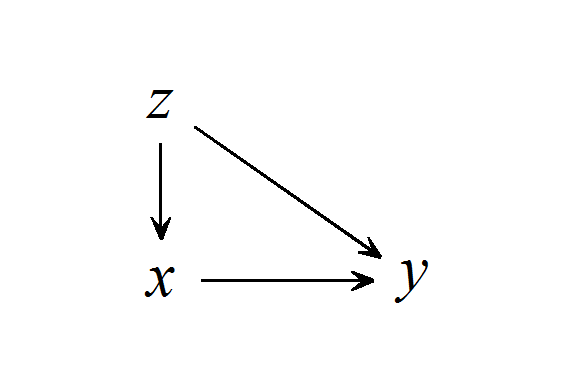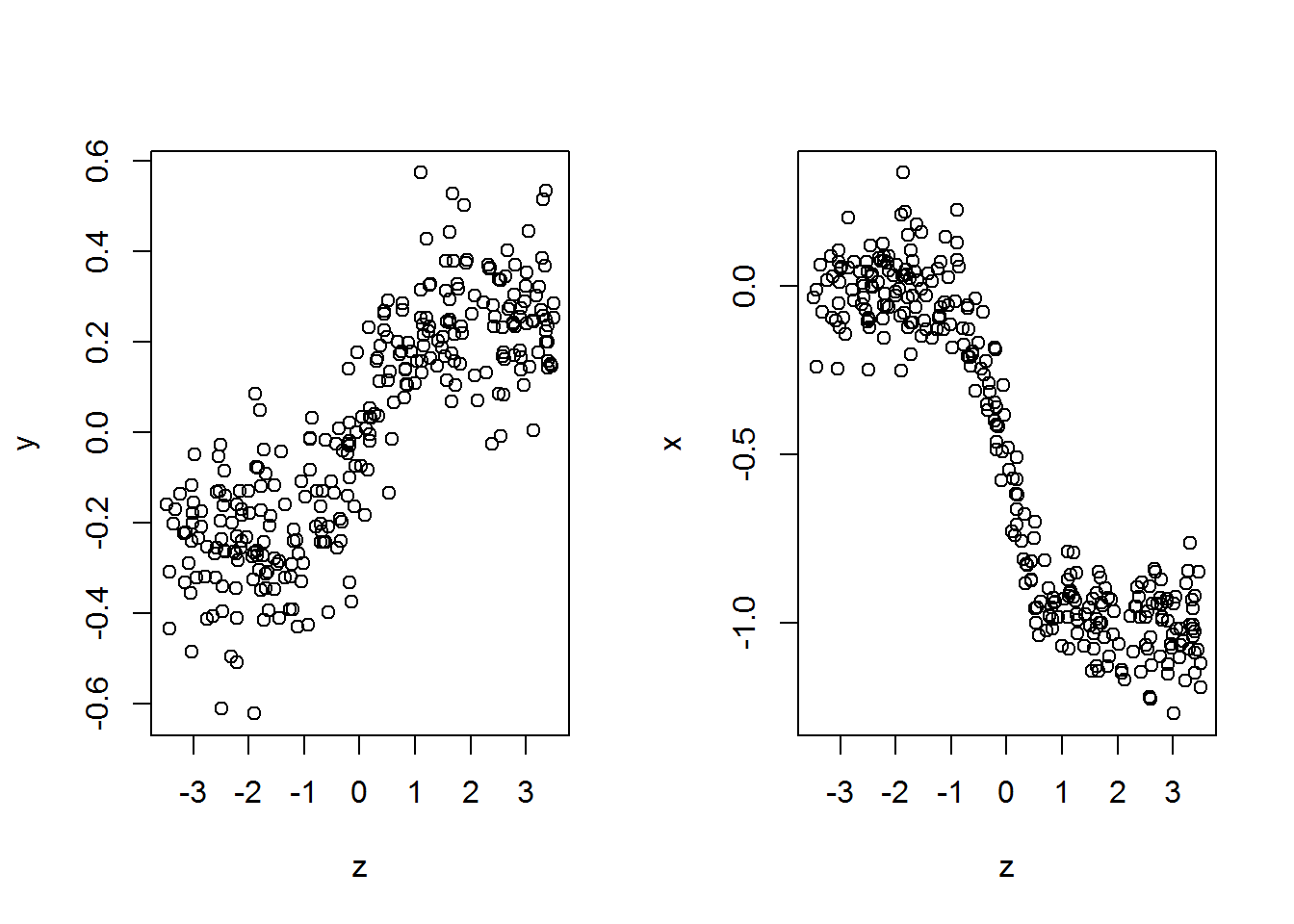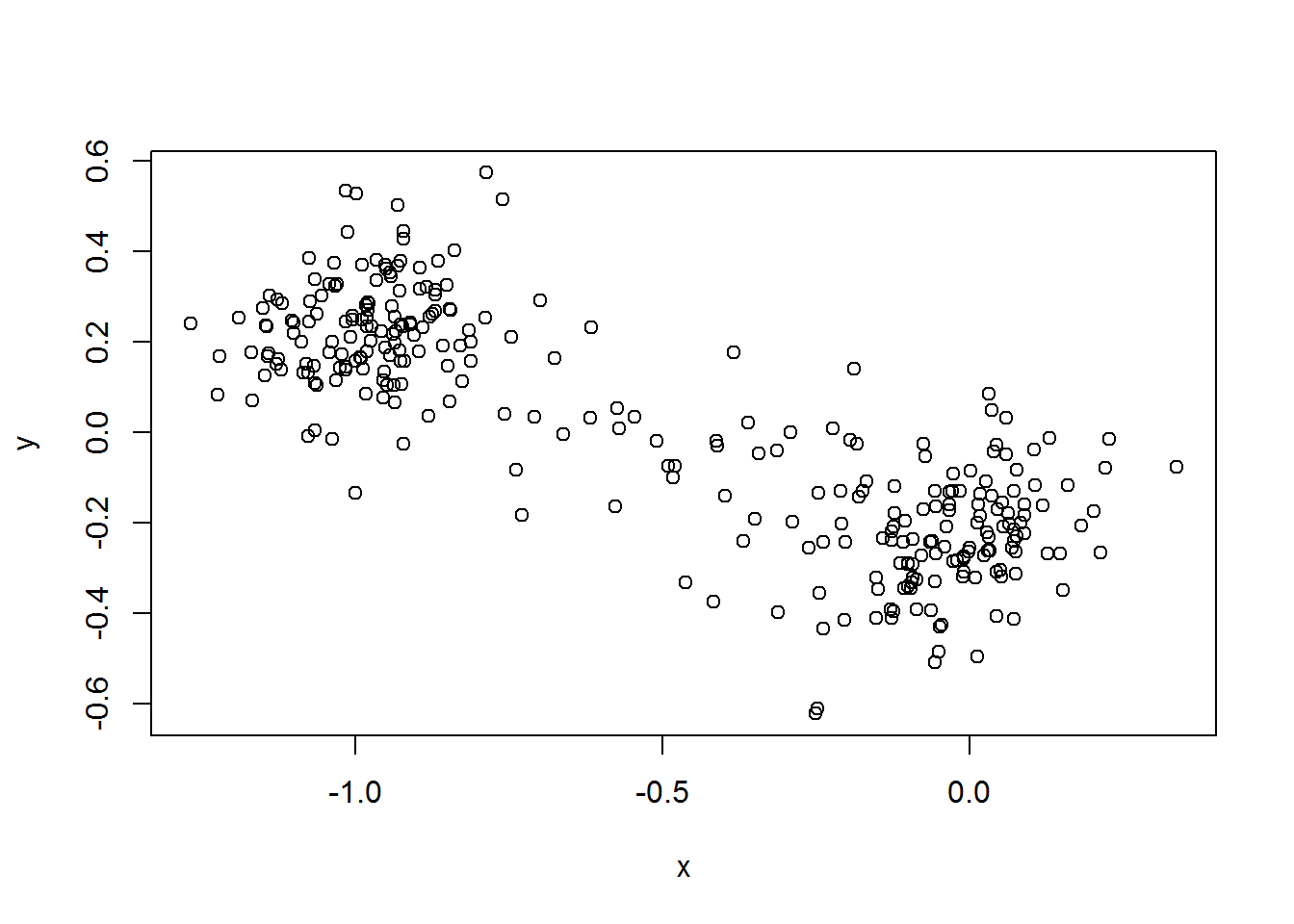## Introduction

I made this intriguing observation when playing with a Gaussian process (GP) example. Initially, I wanted to show that a GP is useful to control for non-linearly related confounding variables. Indeed, it is. But the subtitle about the type-S error was added when I discovered that non-linear confounding can result in data that appears as though it might be reasonably well-handled by a linear model. But in reality, failure to notice and deal with non-linearity with respect to confounders can be detrimental to our inferences.

Type S error: when the estimate has the wrong sign compared with the truth

## The data generating process for the example

### The causal DAG### Traditional notation

Generate $$300$$ data points from a process in which a confounding variable $$z$$ non-linearly causes both $$x$$ and $$y$$ according to a sigmoid function $$f(z)$$, and $$x$$ causes $$y$$ according to a linear function.

$y \sim N(\beta x + f(z), \sigma_y)$

$x \sim N(f(z), \sigma_x)$

The quantity of interest is $$\beta$$, which governs the linear relationship $$x \rightarrow y$$. We set $$\beta$$ to $$0.5$$, and we strive to recover this value in the analysis.

$\beta = 0.5$

$$\sigma_y$$ and $$\sigma_x$$ are the standard deviations of the noise terms for the generation of $$x$$ and $$y$$, and are both set to $$0.1$$.

$\sigma_y = \sigma_x = 0.1$

The logistic function, which I refer to as $$f(z)$$ is defined with an otherwise not-shown vector of parameters $$\mathbf{p}$$.

$f(z,\mathbf{p}) = \frac{p_1}{1+e^{-p_2(z - p_3)}}$

$$\mathbf{p}$$ is a vector of three parameters for the logistic function, and varies depending on whether I’m generating $$x$$ or $$y$$.

### The R code to generate the data

# plant a cool seed for the sake of reproducibility
set.seed(1337)

# sample size
n <- 300

# true beta coefficient for x -> y
beta <- 0.5

# define logistic function for non-linear confounding
f <- function(x, p) {
p / (1 + exp(-p * (x - p)))
}

## generate the data
# d will be our data frame, z is the confounder
d <- data.frame(z=runif(n, min=-3.5, max=3.5))

# x is the independent var, non-linearly caused by
# the confounder plus noise
d$x <- f(d$z, c(-1,3,0)) + rnorm(nrow(d), sd=.1)

# y is the dependent var, non-linearly caused by
# z and linearly caused by x plus noise
d$y <- as.vector(scale( f(d$z, c(1,3,0)) + beta*d\$x + rnorm(nrow(d), sd=.1),
center=T, scale=F))

# check data
head(d)
##            z           x           y
## 1  0.5342508 -1.00068662 -0.13483070
## 2  0.4531949 -0.87473019  0.26065765
## 3 -2.9820684  0.05920894 -0.04914822
## 4 -0.3229406 -0.28820588 -0.19874263
## 5 -0.8870452  0.07709126 -0.08404624
## 6 -1.1807778 -0.06177889 -0.24031871

## Analysis

### Visualize, as one always would

Let’s dive right into the analysis. First, visualize the relationships involving the confounder $$z$$

## plot y across z, and separately x across z
with(d, {
par(mfrow=c(1,2))
plot(z, y)
plot(z, x)
})I see some non-linearity in these relationships. But the relationships maintain their direction throughout (i.e., no A or U shaped relationships here). So maybe it’s safe to assume they are approximately linear functions?

Visualize the relationship between $$x$$ and $$y$$

with(d, {
plot(x, y)
})This looks problematic because we defined the relationship between $$x$$ and $$y$$ to be positive, but this looks negative. That said, I’m sure most of us have heard of Simpson’s paradox, the crux of which is that a failure to control for confounding variables could bias estimates, perhaps enough to generate a type-S error.

### Fit some linear models

An admittedly very brief look at the literature turned up an article titled Examining the Error of Mis-Specifying Nonlinear Confounding Effect With Application on Accelerometer-Measured Physical Activity. The abstract concludes: “For continuous outcomes, confounders with nonlinear effects can be adjusting [sic] with a linear term.”

Can we recover the true causal relationship $$x \rightarrow y$$ using a linear model? First, we’ll fit the model assuming $$y$$ is a simple linear function of $$x$$ and some noise.

## fit an OLS model of y ~ x (no confounder added)
ans1 <- lm(y ~ x, data=d)
summary(ans1)
##
## Call:
## lm(formula = y ~ x, data = d)
##
## Residuals:
##      Min       1Q   Median       3Q      Max
## -0.50584 -0.08590 -0.00032  0.09114  0.45291
##
## Coefficients:
##             Estimate Std. Error t value Pr(>|t|)
## (Intercept) -0.22662    0.01249  -18.14   <2e-16 ***
## x           -0.44145    0.01801  -24.52   <2e-16 ***
## ---
## Signif. codes:  0 '***' 0.001 '**' 0.01 '*' 0.05 '.' 0.1 ' ' 1
##
## Residual standard error: 0.1455 on 298 degrees of freedom
## Multiple R-squared:  0.6685, Adjusted R-squared:  0.6674
## F-statistic:   601 on 1 and 298 DF,  p-value: < 2.2e-16

The coefficient for $$x$$ ($$\hat\beta$$ hereafter) is way off base. If we took this to be a reasonable estimate, we’d certainly be making a type-S error. But really, we didn’t expect that to work correctly, because we know we have a confounding variable $$z$$ involved. So let’s try again with $$z$$ included.

## fit an OLS model including the confounder
ans2 <- lm(y ~ x+z, data=d)
summary(ans2)
##
## Call:
## lm(formula = y ~ x + z, data = d)
##
## Residuals:
##      Min       1Q   Median       3Q      Max
## -0.44714 -0.08950  0.00022  0.09074  0.45628
##
## Coefficients:
##              Estimate Std. Error t value Pr(>|t|)
## (Intercept) -0.103188   0.021798  -4.734 3.42e-06 ***
## x           -0.191331   0.040929  -4.675 4.47e-06 ***
## z            0.062969   0.009395   6.702 1.03e-10 ***
## ---
## Signif. codes:  0 '***' 0.001 '**' 0.01 '*' 0.05 '.' 0.1 ' ' 1
##
## Residual standard error: 0.1359 on 297 degrees of freedom
## Multiple R-squared:  0.7121, Adjusted R-squared:  0.7101
## F-statistic: 367.3 on 2 and 297 DF,  p-value: < 2.2e-16

Nope! $$\hat\beta$$ is still way off. The sign is still wrong (type S error), even if we control for the confounder $$z$$!

## Intermediate discussion

Why am I sufficiently shocked to warrant the “!” in the previous sentence? This is, after all, a post about a non-linear confounder, which we built into the data, and which we did not properly capture in the linear model.

As Stephen Martin pointed out when looking at a draft of this post, it’s not at all clear from the plotted data that one needs to be really worried about the non-linearity of the relationships involving the confounder $$z$$. I mean, maybe a subgroup of you analysts out there knew this was coming. But nothing I’ve ever been taught, in conjunction with the plotted data, would have led me to think …

oh wow, we’re totally going to blow our inference if we don’t do something about that non-linearity.

I might think …

well, I see some non-linearity in there, and I can imagine it creating some bias, or even a type I or type II error, but at least this linear model, controlling for the confounder, will give me an idea of what I’m looking at.

Apparently, it may not even come close!

## Conclude

I want to reiterate what I said in the intermediate discussion. I think a typical applied analyst may look at the data plots I presented and believe a linear model accounting for the confounder $$z$$ would be a totally reasonable first go, if not a totally reasonable full solution. And this post is meant to suggest “maybe not.”